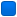###Author Topic: Problems TinyPy scripting.  (Read 2549 times)

#### eightcore

• Newbie
•• Posts: 12##### Problems TinyPy scripting.
« on: January 04, 2013, 08:07:29 PM »
Hello community.

Code: [Select]
`#PY  <- Needed to identify ##--automatically built--adm = Avidemux()adm.loadVideo("")adm.clearSegments()adm.addSegment(0, 0, 7700000)adm.markerA = 0adm.markerB = 7700000adm.videoCodec("x264", "general.params=CBR=150000", "general.threads=99", "general.fast_first_pass=True", "level=31", "vui.sar_height=1", "vui.sar_width=1", "MaxRefFrames=2", "MinIdr=100", "MaxIdr=500", "i_scenecut_threshold=40", "MaxBFrame=2", "i_bframe_adaptive=0", "i_bframe_bias=0", "i_bframe_pyramid=0", "b_deblocking_filter=False", "i_deblocking_filter_alphac0=0", "i_deblocking_filter_beta=0", "cabac=True", "interlaced=False", "analyze.b_8x8=True", "analyze.b_i4x4=False", "analyze.b_i8x8=False", "analyze.b_p8x8=False", "analyze.b_p16x16=False", "analyze.b_b16x16=False", "analyze.weighted_pred=0", "analyze.weighted_bipred=False", "analyze.direct_mv_pred=0", "analyze.chroma_offset=0", "analyze.me_method=0", "analyze.mv_range=16", "analyze.subpel_refine=7", "analyze.chroma_me=False", "analyze.mixed_references=False", "analyze.trellis=1", "analyze.psy_rd=0.000000", "analyze.psy_trellis=0.000000", "analyze.fast_pskip=True", "analyze.dct_decimate=False", "analyze.noise_reduction=0", "analyze.psy=True", "analyze.intra_luma=21", "analyze.inter_luma=21", "ratecontrol.rc_method=0", "ratecontrol.qp_constant=0", "ratecontrol.qp_min=0", "ratecontrol.qp_max=0", "ratecontrol.qp_step=0", "ratecontrol.bitrate=0", "ratecontrol.vbv_max_bitrate=0", "ratecontrol.vbv_buffer_size=0", "ratecontrol.vbv_buffer_init=0", "ratecontrol.ip_factor=0.000000", "ratecontrol.pb_factor=0.000000", "ratecontrol.aq_mode=0", "ratecontrol.aq_strength=0.000000", "ratecontrol.mb_tree=False", "ratecontrol.lookahead=0")adm.addVideoFilter("changeFps", "oldMode=0", "oldFpsDen=1000", "oldFpsNum=30000", "newMode=0", "newFpsDen=1000", "newFpsNum=23940")adm.audioClearTracks()adm.setContainer("AVI", "odmlType=0")`
With this script I convert MOV-files from my Camera to AVI and x264 and change the framerate from 30.0 to about 24.
This script doesn't work anymore. Not long ago, it worked perfectly. Conversion was in progress, I could see the file got bigger and bigger. Today, it doesn't convert the video. CPU usage never exceeds 0.1 percent. It seems that it only copies the mov file and renames it to .avi. File size of the source file and the "converted" one is not identical. About 140 KiB difference. The codec is the same one as before conversion, framerate is still 30 fps.
If i start Avidemux GUI manually and apply exactly the same settings as seen in the script, it works.

Can somone help me?

#### mean

• Global Moderator
• Hero Member
••• Posts: 10974##### Re: Problems TinyPy scripting.
« Reply #1 on: January 05, 2013, 07:36:00 AM »
1-
It might be due to copy/paste by a return is missing
should be
..................=1000", "newFpsNum=23940")

2- a line must no exceed ~256 bytes so you have to break the long videoCode into several lines

3- What version of avidemux are you using ?

#### eightcore

• Newbie
•• Posts: 12##### Re: Problems TinyPy scripting.
« Reply #2 on: January 05, 2013, 06:45:07 PM »
I'm using 2.6.1.

This (256 bytes per line) solved it. Thank you very much!
« Last Edit: January 05, 2013, 07:56:57 PM by eightcore »